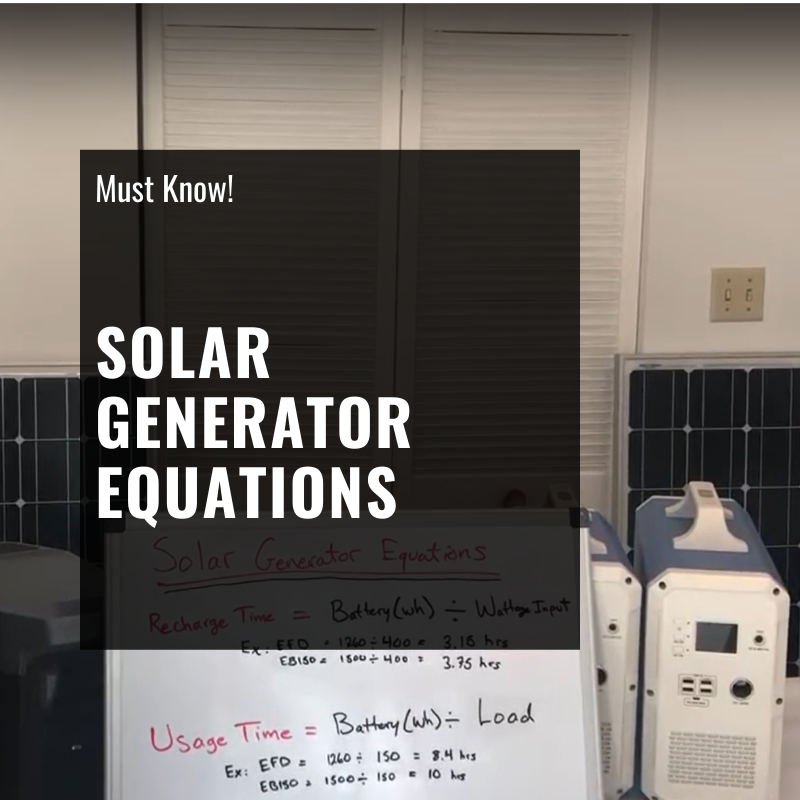MUST KNOW Off-Grid Solar Sizing Equations: Calculate EXACT Run Times - ShopSolar.com

# MUST KNOW Solar Sizing Equations: Learn How to Calculate EXACT Battery Bank Recharge Time & Run TimeWondering how long a solar generator / solar battery will last in hours? Or how long it will take to charge a solar generator / solar battery bank from solar panels?

The video below explains the two most important solar sizing equations you need to know when shopping for ANY solar generator / off-grid (battery based) solar kit. Once you understand these two solar sizing equations, you will know how to choose the best solar generator for your needs.

The 2 most common off-grid solar questions we get asked are:

#1. How long will it take to recharge this solar generator from the sun?

#2. How long will this solar generator last me?

The video explains the exact calculations you need to know in order to figure out the answers to the two questions above. (Keep in mind the same logic applies to bigger off-grid battery banks as well).

Again, to recap the video, these two off-grid solar sizing equations are: